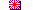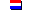[  ]

Norel H van

60 p in Dutch   1992

 Toon Nederlands English Abstract The mathematical model AQ-EC simulates the transport of a solute in flowing groundwater. The model uses the numerical approximation of the finite element method to solve the differential equations. Numerical approximations are subject to deviations like numerical dispersion and instability. Those deviations can be analyzed by comparing the results of the numerical calculations and the analytical solutions. The model AQ-EC was checked using the analytical model TRANS for the processes convection and dispersion. The analytical "travelling wave" equation was applied to verify the calculations for the processes convection, dispersion and non-linear adsorption.  RIVM - Bilthoven - the Netherlands - www.rivm.nlDisplay English Rapport in het kort Abstract niet beschikbaar  RIVM - Bilthoven - Nederland - www.rivm.nl( 1992-05-31 )